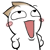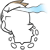12返回列表 发帖
 本帖最后由 freefree2010 于 2018-1-2 04:38 编辑 中4球 3X100=300 中3球 21X10=210 中2球 95X2=190 中700－花500＝200  呵呵，本人到此一游，意外捡钱，甚是惊喜

 完全没中奖,年年如此,亏了,亏了.
 4球 4×100=400 3球 100×10=1000 2球 420×2=840 400+1000+840-2000=240 還可以啦

 5球: 1x1000=1000 4球: 11x100=1100 3球: 97x10=970 2球: 413x2=826 賺18964:100*1=100 3:10*28=280 2:2*159=318 100+280+318=698 702-698=4 虧了一點，還可以、還可以! 祝2018大家事事順利、平安順心~
 4球：6×100＝600 3球：93×10＝930 2球：406×2=812 5球：1000*1=1000 600+930+812+1000-2000=1342 口算还行吧。

BL:南北杂货，疯狗加三，奇幻异典，生随死殉，安乐死，民国之文豪

 怎么感觉今年的名单比以前的短，是买的人变少了吗，咱们论坛的人变少了？看余额应该是赚了一点零头，懒得计算了中4球： (90)x100 =900 中3球：(70)x10 =700 中2球：(440) x2 = 880 Total (2480) - Cost (2000) = 480 小赚，就是数字，哈哈哈哈
 4球 10×100=1000 3球 81×10=810 2球 452×2=904 小赚 714
 一个五球都没中啊中三球：(2) x 10 = 20 中两球：(2) x 2 = 4 Total: 24 - 15 (COST) = 9 (⊙o⊙)…试试手也赚了一点点已经很开森
 本帖最后由 201208 于 2018-1-5 11:27 编辑 6x100=600 81x10=810 422x2=844 600+810+844=2254 赚了254 ……………… 何止少一点人！少好多！这里还有一个人多个号的！人更少！

 4球：6×100=600 3球：97×10=970 2球：444×2=888 600+970+888=2458，赚458